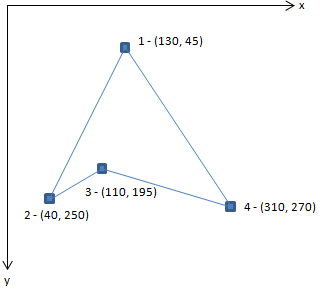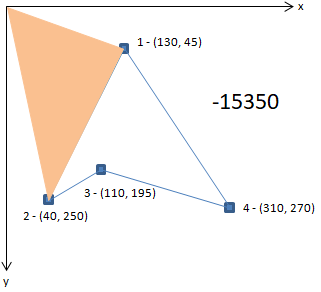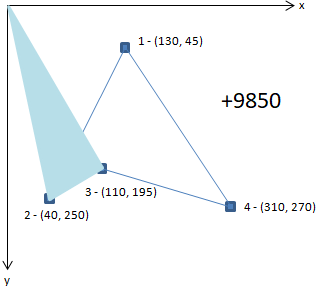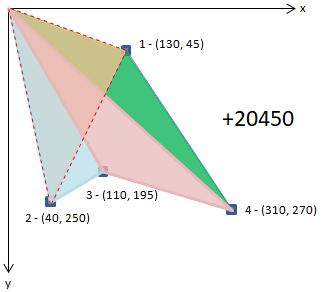﻿ Sunshine's Homepage - Polygon Area Javascript
Polygon area (Javascript)

### Description

This tiny application allows to specify the vertices of a polygon and to calculate its area.
Note that it must be a simple polygon to work correctly, without holes and overlaps.

### Usage information

• By clicking with the left mouse button on the grey rectangle above, a new polygon vertice can be added.
• By clicking with the right mouse button on an existing point, it is removed.
• It is possible to drag an existing point with the left mouse button to change its position.
• The area can only be calculated if the polygon is closed. Close a polgon by clicking the first vertice of the polygon with the left mouse button.
• The option to calculate the orientation of a the polygon (clockwise or counter-clockwise) is just an bonus option and is not required to the area calculation.

## Notes for polygon area calculation

The Gauss's area formula is used to calculate the area of the simple polygon.
The main idea and steps are as follows:

• For each edge AB of the polygon and origin point C, calculate the area of the triangle ABC using the cross prodcut.
• The area of such a triangle could be positive or negative, depending on the order of the vertices. (Clock-wise: negative, counter-clockwise: positive).
• An triangle having the edge on the right side might overlap with a triangle having an edge of the right side. However due to the orientation one area will be positive while the other area will be calculated negative. The triangle area on the left of the polygon will be counted twice, but due to the different signs it will be cancelled out. It remains only the area of the triangle inside the polygon that contributes to the total area. (Have a look at the example below that hopefully makes the process clearer!).

So let's do an example step-by-step:In this example we use the triangle on the left. Note that the vertices are numbered and thus the triangle is defined in counter-clockwise order.The first triangle is formed by vertice 1 (130, 45) and vertics 4 (310 270) with the origin.
Remember the cross product is calculated as ax * by - ay * bx (where a and b are two vectors). We always use the vectors from the origin to the current two vertices. The cross product here is:
130 * 270 - 45 * 310 = 21150.
Note that the area of the triangle is half the cross product, thus 10575.Next triangle is formed by vertice 2 (40, 250) and vertice 1 (130, 45) with the origin.

So the cross product is:
40 * 45 - 250 * 130 = -30700.
Half of -30700 is -15350.

Total area of the example triangle until now is:
10575 - 15350 = -4775Next triangle is formed by vertice 3 (110, 195) and vertice 2 (40, 250) with the origin.

So the cross product is:
110 * 250 - 195 * 40 = 19700.
Half of 19700 is 9850.

Total area of the example triangle until now is:
10575 - 15850 + 9850 = 5075Last triangle is formed by vertics 4 (310 270) and vertice 3 (110, 195) with the origin.

So the cross product is:
310 * 195 - 270 * 110 = 30750.
Half of 30750 is 15375.

Total area of the example triangle until now is:
10575 - 15850 + 9850 + 15375 = 20450Summarized, the area of the rot-dotted triangle is included by the steps of the green, blue and purple triangles. However, it is competely subtracted in the step with the orange triangle: the sign is of the cross product is the opposite because of the direction and order of the vertices, so the remaining calculated area matches exactly the area of the target triangle.

Hope you liked it!

Sunshine2k, July 2k17

### History

2016/07/14: Initial release.